# 玻色-爱因斯坦凝聚中的涡旋晶格形成模拟

2020年 11月 17日

### “薛定谔方程”物理场接口

COMSOL Multiphysics 的半导体模块具有一个薛定谔方程 物理场接口。在一个最简单的应用示例中，它描述了非相对论粒子在势能图景影响下的动力学（参考文献1）。它可以使用包络函数近似模拟量子约束固态系统，例如量子阱、量子线和量子点（参考文献2）。在本博客文章中，我们将演示如何将薛定谔方程转换成 Gross–Pitaevskii 方程（参考文献3和4），以及如何将其用于模拟玻色凝聚系统。

### 涡旋晶格形成实验

Madison、Chevy、Bretin 和 Dalibard 于 2001 年发表的论文（参考文献5）中展示了一系列引人注意的图像（论文中的图3），它生动地证明了在旋转激光场的作用下，玻色-爱因斯坦凝聚原子云中涡旋晶格的成核和形成。在同一图中，他们还绘制了椭圆率 |\tilde\alpha| 的时间演化图（请参阅下面的公式（7）），显示了当进入涡旋晶格的低能态之前，该系统经历了一段明显的动力学不稳定期。先是初始振荡，随后 |\tilde\alpha| 坍塌至接近零。(1)

V_{trap}=m \omega_t^2(x^2+y^2)/2+m\omega_z^2 z^2/2

(2)

V_{laser}=m\omega_t^2(\epsilon_X X^2+\epsilon_Y Y^2)/2

(3)

\epsilon\equiv(\omega_X^2-\omega_Y^2)/(\omega_X^2+\omega_Y^2)=(\epsilon_X-\epsilon_Y)/(2+\epsilon_X+\epsilon_Y)

(4)

\bar\omega \equiv \sqrt{(\omega_X^2+\omega_Y^2)/2}=\omega_t\sqrt{(2+\epsilon_X+\epsilon_Y)/2}

(5)

\rho_{TF}=max\left[0 , \frac{\mu_{TF}}{g}\left(1-\frac{X^2}{R_X^2}-\frac{Y^2}{R_Y^2}-\frac{z^2}{R_z^2}\right)\right]

(6)

\alpha\equiv\Omega\frac{R_X^2-R_Y^2}{R_X^2+R_Y^2}

(7)

\tilde\alpha\equiv\alpha/\bar\omega=\tilde\Omega\frac{R_X^2-R_Y^2}{R_X^2+R_Y^2}

### 建模理论

(8)

(i-\gamma)\hbar\frac{\partial\psi}
{\partial t}
=\left[-\frac{\hbar^2}
{2m}\nabla^2+V_{trap}+V_{laser}+g|\psi|^2-\mu-\Omega L_z\right]\psi

Choi 等人（参考文献3）提供了估算阻尼系数 \gamma 的公式：

(9)

\gamma\approx\frac{4m(a k T)^2}
{\pi\hbar^3}
e^{2\mu/k T}\frac{\mu}
{k T}K_1\left(\frac{\mu}{k T}
\right)/\bar\omega

Thomas-Fermi 近似可以很好地估算许多重要参数，例如阻尼系数 \gamma。圆柱形对称势阱中的峰值密度和尺寸参数总结如下：

(10)

\begin{align*}
\rho_0\equiv\frac{\mu_{TF}}{g} &= \frac{15N}{8\pi R_r^2 Rz} \\
R_r &= \left(\frac{15g \omega_z N}{4\pi m \bar\omega^3}\right)^{1/5}\\
R_z &= \left(\frac{15g \bar\omega^2 N}{4\pi m \omega_z^4}\right)^{1/5}
\end{align*}

### 模拟涡旋晶格的形成

#### 模型参数

Gross-Pitaevskii 方程（8）可以使用半导体模块中的薛定谔方程 物理场接口直接实现。在构建模型时，我们将模型参数与参考文献1中的实际实验条件进行匹配，以使模拟的时间演变与已发布的数据完全吻合。

epst=0.032*step1((t+tau)/tau)*(1-step1((t-t_off)/tau))

epsX=(epst+sqrt(0.03*0.09+epst^2-0.03*0.09*epst^2))/(1-epst)

epsY=(-epst+sqrt(0.03*0.09+epst^2-0.03*0.09*epst^2))/(1+epst)

(11)

L=\frac{N}{\rho_0 \frac{\pi}{2} R_r^2}

#### 初始静止状态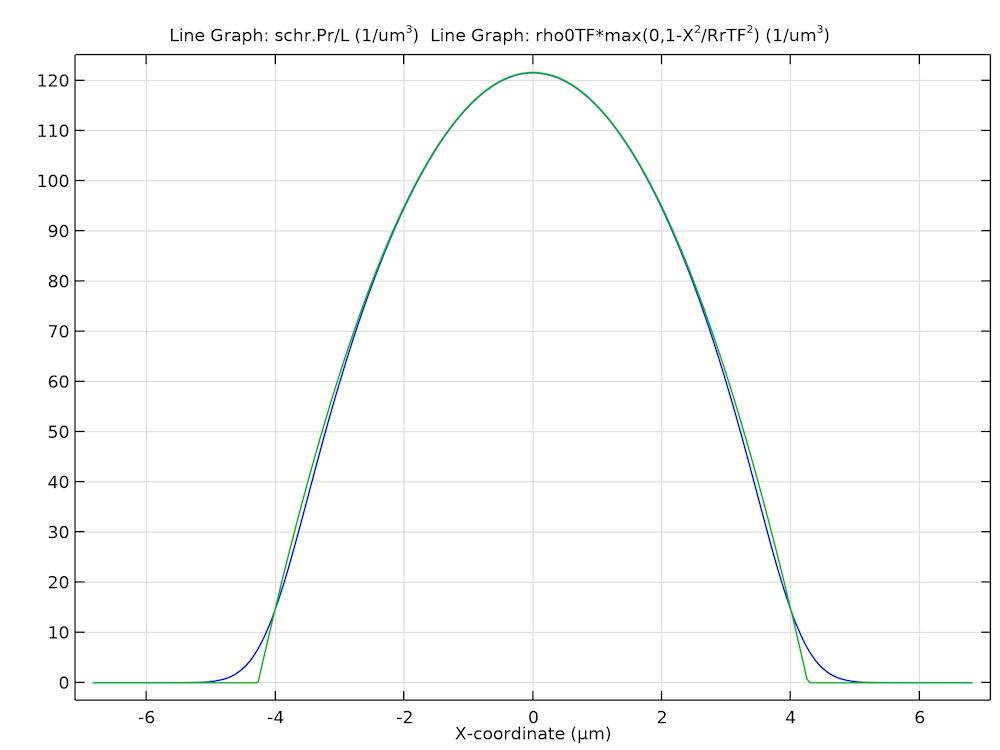X 轴上的粒子密度分布的计算稳态解（蓝色）和通过 Thomas–Fermi 近似求出的一个稳态解（绿色）。

#### 旋转框架、耗散和归一化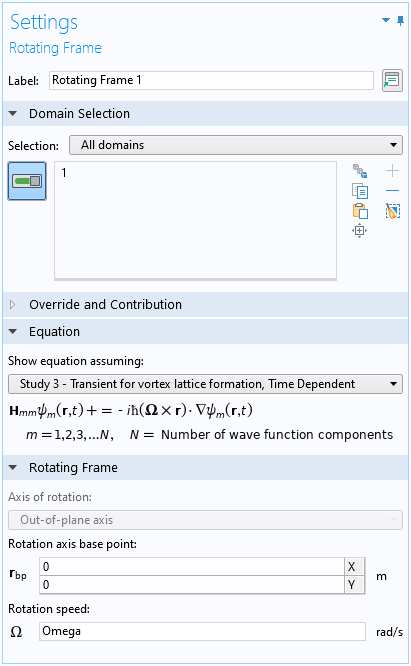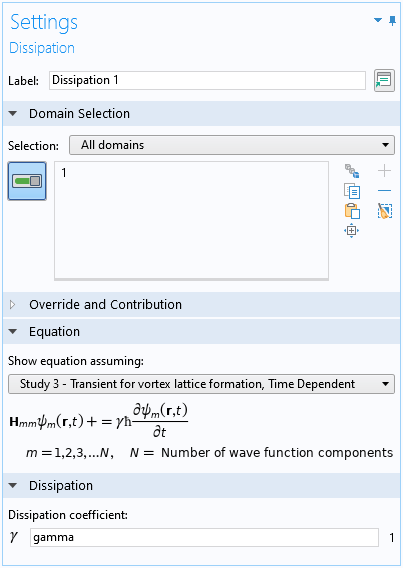#### 动态不稳定性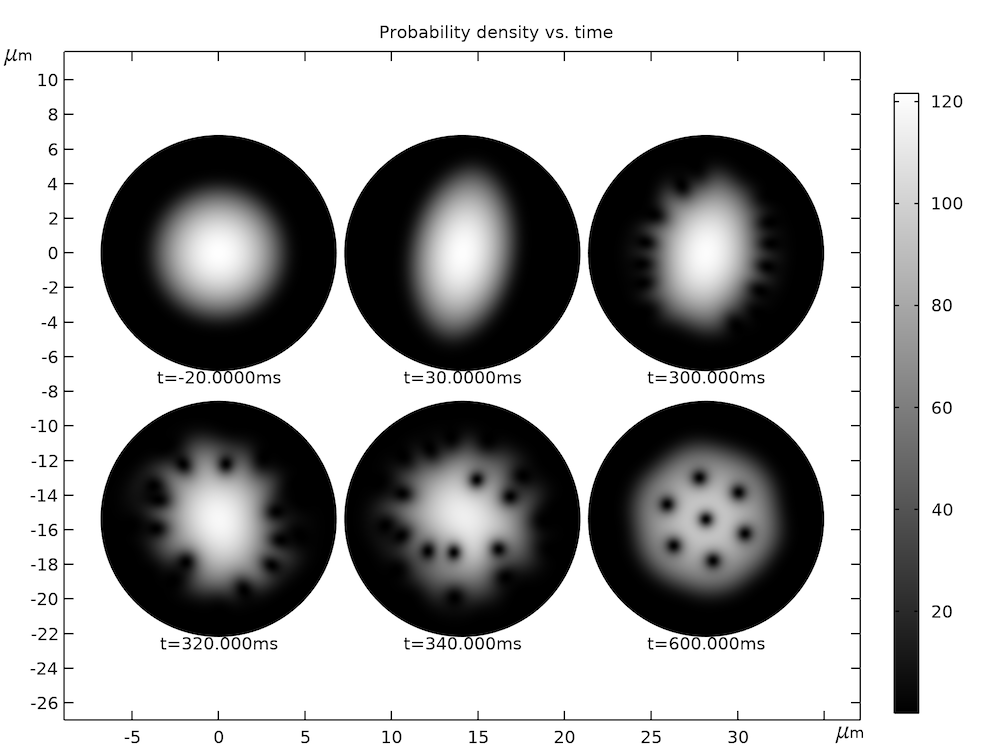### 结果分析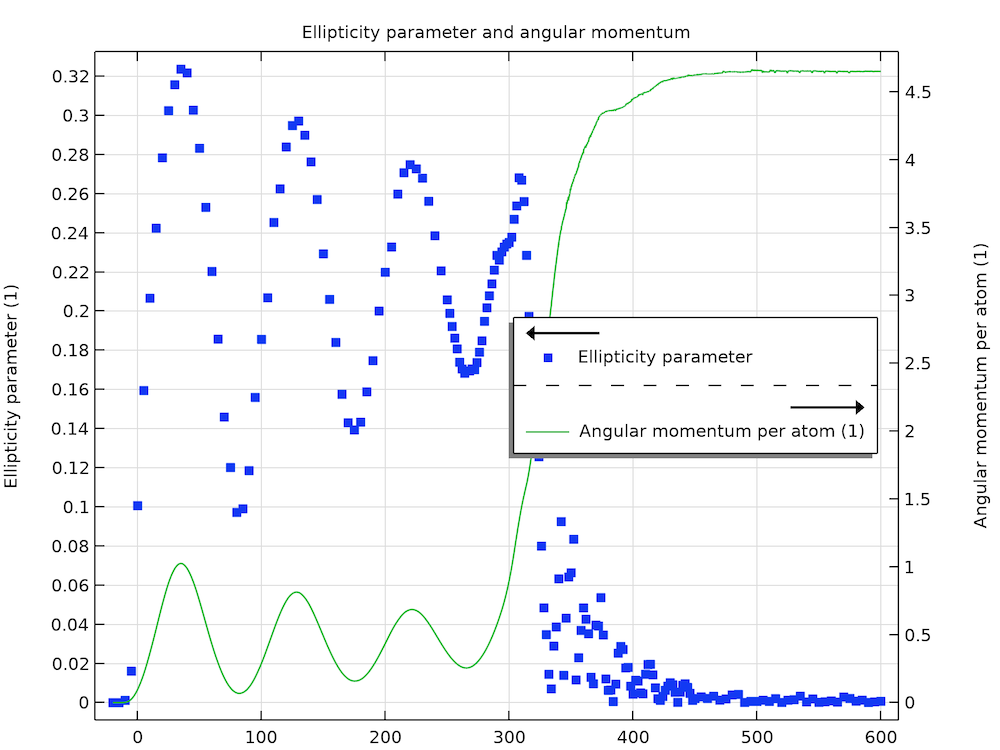#### 优化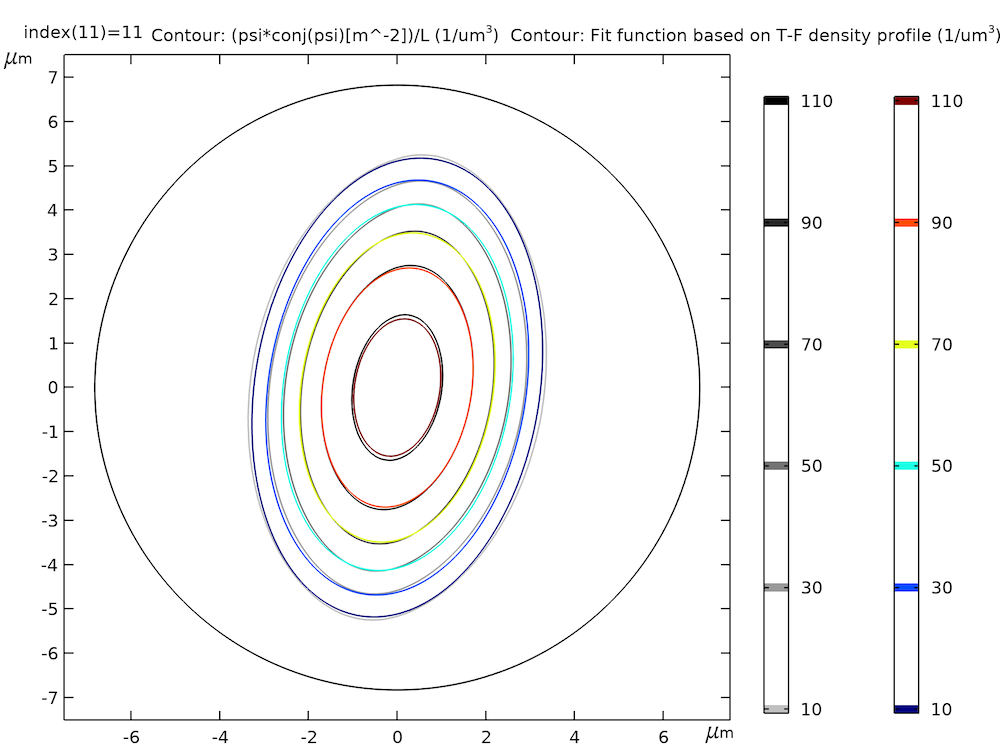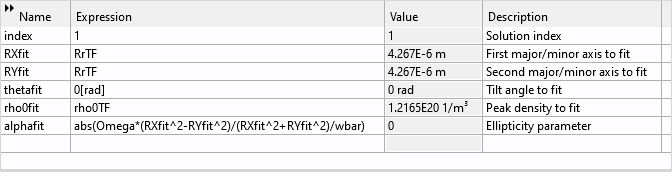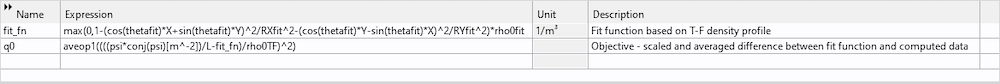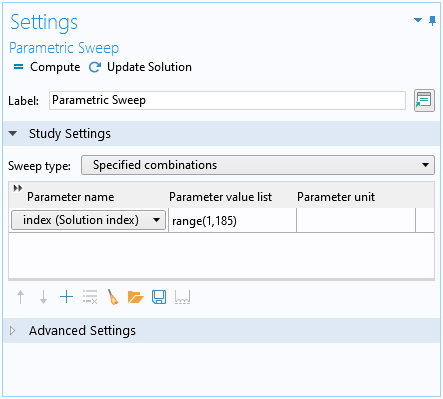### 结语

1. 1. L. I. Schiff, 《Quantum Mechanics》, McGraw-Hill, ed. 3, 1968.
2. 2. P. Harrison, 《Quantum Wells, Wires and Dots》, Wiley, ed. 3, 2009.
3. 3. E.P. Gross, “Structure of a quantized vortex in boson systems”, Il Nuovo Cimento, vol. 20, no. 3, pp. 454–457, 1961.
4. 4. L.P. Pitaevskii, “Vortex lines in an imperfect Bose gas”, Sov. Phys. JETP, vol. 13, no. 2, pp 451–454, 1961.
5. 5. K. W. Madison, F. Chevy, V. Bretin, and J. Dalibard, “Stationary States of a Rotating Bose-Einstein Condensate: Routes to Vortex Nucleation”, Phys. Rev. Lett., 86, 4443, 2001.
6. 6. M. Tsubota, K. Kasamatsu, and M. Ueda,《“Vortex lattice formation in a rotating Bose-Einstein condensate”,, Phys. Rev., A 65, 023603 (2002).
7. 7. S. Choi, S. A. Morgan, and K. Burnett, “Phenomenological damping in trapped atomic Bose-Einstein condensates”, Phys. Rev., A 57, 4057, 1998.